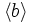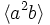# Generalized quaternion group:Q16

View a complete list of particular groups (this is a very huge list!)[SHOW MORE]

## Definition

The group$Q_{16}$, sometimes termed the generalized quaternion group of order$16$, is a generalized quaternion group. It can be described by the following presentation:$G := \langle a,b,c \mid a^4 = b^2 = c^2 = abc \rangle$.

Note that$c = ab = ba^{-1}$ from these relations, and$bab^{-1} = a^{-1}$. This in turn forces that$b^2 = b(b^2)b^{-1} = ba^4b^{-1} = a^{-4} = b^{-2}$, forcing$b^2 = a^4 = c^2 = abc$ to have order two. We shall denote this element of order two, which is clearly central, as$z$.

We can thus use an alternative presentation that requires only two generators:$G := \langle a,b \mid a^4 = b^2 = abab \rangle$

## Arithmetic functions

Want to compare and contrast arithmetic function values with other groups of the same order? Check out groups of order 16#Arithmetic functions
Function Value Similar groups Explanation for function value
underlying prime of p-group 2
order (number of elements, equivalently, cardinality or size of underlying set) 16 groups with same order
prime-base logarithm of order 4 groups with same prime-base logarithm of order
max-length of a group 4 max-length of a group equals prime-base logarithm of order for group of prime power order
chief length 4 chief length equals prime-base logarithm of order for group of prime power order
composition length 4 composition length equals prime-base logarithm of order for group of prime power order
exponent of a group 8 groups with same order and exponent of a group | groups with same exponent of a group cyclic subgroup of order 8.
prime-base logarithm of exponent 3 groups with same order and prime-base logarithm of exponent | groups with same prime-base logarithm of order and prime-base logarithm of exponent | groups with same prime-base logarithm of exponent
nilpotency class 3 groups with same order and nilpotency class | groups with same prime-base logarithm of order and nilpotency class | groups with same nilpotency class
derived length 2 groups with same order and derived length | groups with same prime-base logarithm of order and derived length | groups with same derived length the derived subgroup is contained in the cyclic subgroup and is hence abelian
Frattini length 3 groups with same order and Frattini length | groups with same prime-base logarithm of order and Frattini length | groups with same Frattini length
minimum size of generating set 2 groups with same order and minimum size of generating set | groups with same prime-base logarithm of order and minimum size of generating set | groups with same minimum size of generating set
subgroup rank of a group 2 groups with same order and subgroup rank of a group | groups with same prime-base logarithm of order and subgroup rank of a group | groups with same subgroup rank of a group
rank of a p-group 1 groups with same order and rank of a p-group | groups with same prime-base logarithm of order and rank of a p-group | groups with same rank of a p-group all abelian subgroups are cyclic.
normal rank of a p-group 1 groups with same order and normal rank of a p-group | groups with same prime-base logarithm of order and normal rank of a p-group | groups with same normal rank of a p-group all abelian normal subgroups are cyclic.
characteristic rank of a p-group 1 groups with same order and characteristic rank of a p-group | groups with same prime-base logarithm of order and characteristic rank of a p-group | groups with same characteristic rank of a p-group All abelian characteristic subgroups are cyclic.

### Arithmetic functions of a counting nature

Function Value Similar groups Explanation
number of conjugacy classes 7 groups with same order and number of conjugacy classes | groups with same prime-base logarithm of order and number of conjugacy classes | groups with same number of conjugacy classes As$Q_{2^n}, n = 4$:$2^{n-2} + 3 = 2^2 + 3 = 7$
number of equivalence classes under rational conjugacy 6 groups with same order and number of equivalence classes under rational conjugacy | groups with same prime-base logarithm of order and number of equivalence classes under rational conjugacy | groups with same number of equivalence classes under rational conjugacy
number of conjugacy classes of rational elements 5 groups with same order and number of conjugacy classes of rational elements | groups with same prime-base logarithm of order and number of conjugacy classes of rational elements | groups with same number of conjugacy classes of rational elements

## Group properties

### Important properties

Property Satisfied? Explanation Comment
group of prime power order Yes
nilpotent group Yes prime power order implies nilpotent
supersolvable group Yes via nilpotent: finite nilpotent implies supersolvable
solvable group Yes via nilpotent: nilpotent implies solvable
abelian group No$a,b$ don't commute.
metacyclic group Yes$\langle a \rangle$ is cyclic of order eight, quotient group is cyclic of order two.
metabelian group Yes follows from being metacyclic.

### Other properties

Property Meaning Satisfied? Explanation Comment
finite group with periodic cohomology finite group in which every abelian subgroup is cyclic Yes
Schur-trivial group the Schur multiplier is trivial Yes follows from having periodic cohomology
maximal class group finite p-group of class more than one whose class is one less than the prime-base logarithm of order Yes class is 3, prime-base logarithm of order is 4.
UL-equivalent group upper central series and lower central series coincide.
stem group the center is contained in the derived subgroup Yes follows from being a non-abelian UL-equivalent group.
directly indecomposable group nontrivial and cannot be expressed as an internal direct product of nontrivial subgroups Yes
centrally indecomposable group nontrivial and cannot be expressed as a internal central product of proper nontrivial subgroups Yes
splitting-simple group nontrivial and cannot be expressed as an internal semidirect product of nontrivial subgroups Yes

## Subgroups

Further information: Subgroup structure of generalized quaternion group:Q16

1. The trivial subgroup. Isomorphic to trivial group. (1)
2. The center, which is a subgroup of order two, generated by$z = a^4 = b^2 = c^2$. Isomorphic to cyclic group:Z2. (1)
3. The cyclic subgroup of order four generated by$a^2$. Isomorphic to cyclic group:Z4. (1)
4. The four cyclic subgroups of order four, namely:$\langle b \rangle$,$\langle ab \rangle$,$\langle a^2b \rangle$ and$\langle a^3b\rangle$. These come in two conjugacy classes of 2-subnormal subgroups, one conjugacy class comprising$\langle ab \rangle$ and$\langle a^3b \rangle$ and the other comprising$\langle b \rangle$ and$\langle a^2b \rangle$. Isomorphic to cyclic group:Z4. (4)
5. The cyclic subgroup of order eight, generated by$a$. This is characteristic; in fact, it equals the centralizer of commutator subgroup. Isomorphic to cyclic group:Z8. (1)
6. Two quaternion groups of order eight, namely$\langle a^2,b \rangle$ and$\langle a^2, ab \rangle$. Isomorphic to quaternion group. (2)
7. The whole group. (1)

## Subgroup-defining functions

Subgroup-defining function Subgroup type in list Page on subgroup embedding Isomorphism class Comment
Center (2) cyclic group:Z2
Commutator subgroup (3) cyclic group:Z4
Frattini subgroup (3) cyclic group:Z4
Socle (2) cyclic group:Z2
Join of abelian subgroups of maximum order (5) cyclic group:Z8
Join of abelian subgroups of maximum rank (7) whole group
Join of elementary abelian subgroups of maximum order (2) cyclic group:Z2

## GAP implementation

### Group ID

This finite group has order 16 and has ID 9 among the groups of order 16 in GAP's SmallGroup library. For context, there are 14 groups of order 16. It can thus be defined using GAP's SmallGroup function as:

SmallGroup(16,9)

For instance, we can use the following assignment in GAP to create the group and name it$G$:

gap> G := SmallGroup(16,9);

Conversely, to check whether a given group$G$ is in fact the group we want, we can use GAP's IdGroup function:

IdGroup(G) = [16,9]

or just do:

IdGroup(G)

to have GAP output the group ID, that we can then compare to what we want.

### Hall-Senior number

This group of prime power order has order 16 and has Hall-Senior number 14 among the groups of order 16. This information can be used to construct the group in GAP using the Gap3CatalogueGroup function as follows:

Gap3CatalogueGroup(16,14)

WARNING: There is some disagreement between the GAP 3 catalogue numbers and the Hall-Senior numbers for some abelian groups, but it does not affect this group.

For instance, we can use the following assignment in GAP to create the group and name it$G$:

gap> G := Gap3CatalogueGroup(16,14);

Conversely, to check whether a given group$G$ is in fact the group we want, we can use GAP's Gap3CatalogueIdGroup function:

Gap3CatalogueIdGroup(G) = [16,14]

or just do:

Gap3CatalogueIdGroup(G)

to have GAP output the group ID, that we can then compare to what we want.

### Description by presentation

gap> F := FreeGroup(2);
<free group on the generators [ f1, f2 ]>
gap> G := F/[F.1^8,F.2^2*F.1^(-4),F.2*F.1*F.2^(-1)*F.1];
<fp group on the generators [ f1, f2 ]>
gap> IdGroup(G);
[ 16, 9 ]

### Other descriptions

Description Functions used
SylowSubgroup(SL(2,7),2) SylowSubgroup, SL